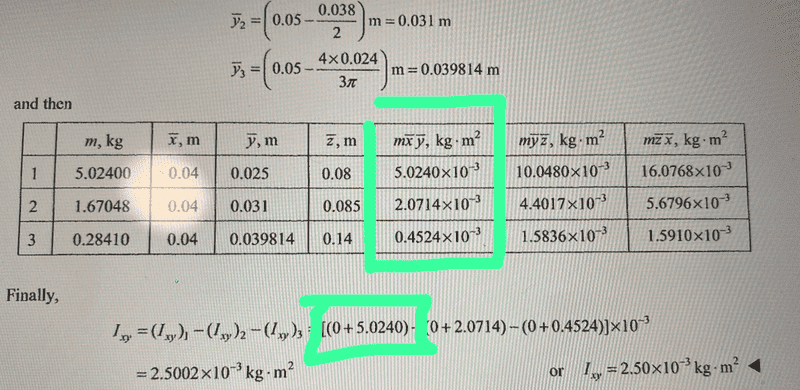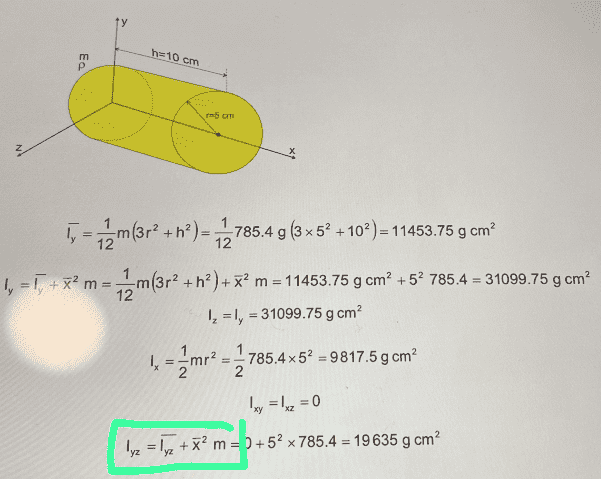# Mass inertia product

• I
Guillem_dlc
TL;DR Summary
How is the mass inertia product calculated? I have two examples and each one uses something different.
How is the mass inertia product calculated? I have two examples and each one uses something different.

Example 1:Example 2: moments and product of inertia of the cylinder